# Memoryless Nonlinearity

Apply amplifier models to complex baseband signal

•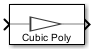Libraries:
Communications Toolbox / RF Impairments and Components

## Description

The Memoryless Nonlinearity block applies amplifier models to a complex baseband signal. Use this block to model memoryless nonlinear impairments caused by signal amplification in the radio frequency (RF) transmitter or receiver. For more information, see Amplifier Model Methods.

## Examples

expand all

This example applies the Saleh method of memoryless nonlinearity to a 16-QAM modulated signal. To show the Saleh model of a power amplifier, the example applies exaggerated levels that are not typical for modern radios.

In this example, the `cm_mnl_saleh_16qam` model 16-QAM modulates a signal containing a complete set of constellation points and passes them to Memoryless Nonlinearity blocks configured to apply the Saleh method with the default AM/AM and AM/PM distortion settings, AM/AM distortion alone, and AM/PM distortion alone. The model includes Constellation Diagram blocks after each Memoryless Nonlinearity block so you can analyze the impact of each impairment on the constellation.In the model, you can click the Plot Transfer Curve Callback Button (Simulink) block to run the `testPA.m` helper function. The `testPA` helper function plots this amplifier transfer curve for the Saleh method in its default configuration to show the nonlinear input-to-output signal. After amplification, plotted constellation points get displaced according to the input-to-output characteristics of the amplifier model. The voltage for each constellation point determines the direction and magnitude of distortion for each point in the constellation.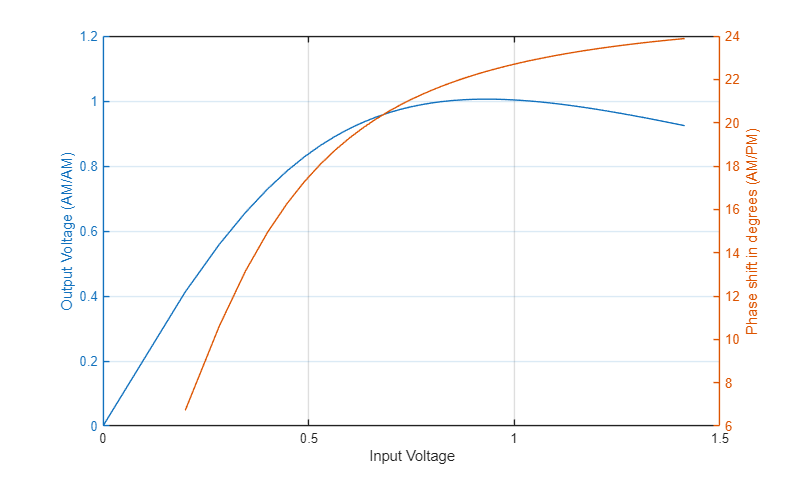This set of constellation diagrams shows the constellation distortion for the amplifier configured to distort the amplitude and phase individually and together.

• The `AMAM` diagram shows the signal with amplitude-to-amplitude distortion but no amplitude-to-phase distortion. AM/AM distortion displaces constellation points radially away from or toward the origin. For the Saleh method and specified operating characteristics, the inner corner constellation points move away from the origin and the outer corner constellation points move toward the origin. The noncorner constellation points move a negligible amount.

• The `AMPM` diagram shows the signal with amplitude-to-phase distortion but no amplitude-to-amplitude distortion. AM/PM distortion causes rotation of constellation points. Constellation points farther from the origin are more displaced than points closer to the origin.

• The `AMAM and AMPM` diagram shows the signal with amplitude-to-amplitude distortion and amplitude-to-phase distortion. In this diagram, the constellation points move away from or toward the origin and rotate counter-clockwise.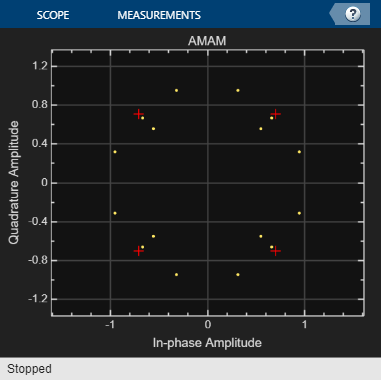This scatter plot of the constellation points shows points displaced by AM/AM distortion only. The `scatterplot` function used here plots constellation point locations labeled to highlight the relative distance each point has moved from the ideal constellation point location. To analyze the magnitude and direction each constellation point moved, you would need to replot the input and output characteristic curve for the current operating characteristics.To explore the model try adjusting settings for the Memoryless Nonlinearity blocks to:

• Apply different impairment model methods.

• Apply different levels of impairments.

• Update the `testPA.m` helper function to plot and analyze input and output characteristics at nondefault Memoryless Nonlinearity block settings.

This example applies RF impairments to a signal modulated by the differential quadrature phase shift keying (DQPSK) method. To show the RF impairments, the example applies exaggerated levels that are not typical levels for modern radios.

In this example, the `slex_rcvrimpairments_dqpsk` model DQPSK-modulates a random signal and applies various RF impairments to the signal. The model uses impairment blocks from the RF Impairments library. The `InitFun` callback function initializes simulation variables. For more information, see Model Callbacks (Simulink).

After the impairment blocks, the signal forks into two paths. One path applies DC blocking, automatic gain control (AGC), and I/Q imbalance compensation to the signal before demodulation. The signal on the correction path is adjusted by the DC Blocker, AGC, and I/Q Imbalance Compensator blocks. Because the signal is DQPSK modulated, no carrier synchronization is required. The second path goes directly to demodulation. After demodulation, an error rate calculation is performed on both signals. The model includes Constellation Diagram blocks after modulation, before correction, and after correction so that you can analyze the constellation.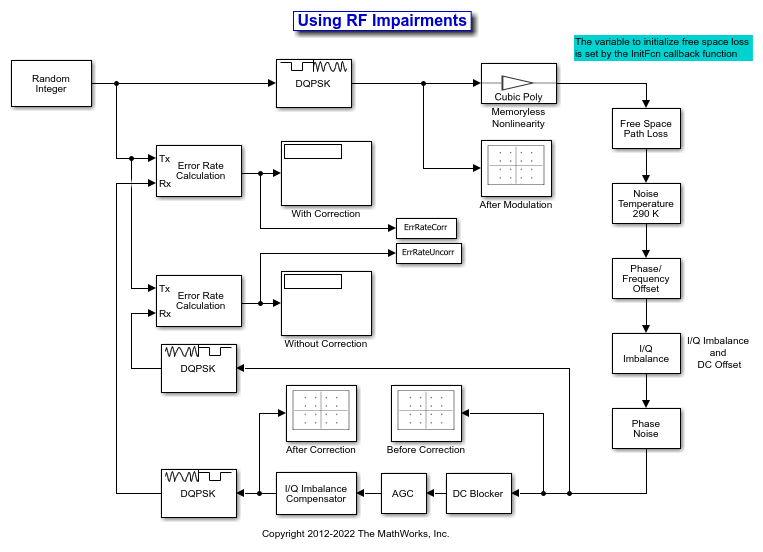When the model runs, constellation diagrams plot the signal at these stages in the simulation:

• The `After Modulation` constellation diagram shows the reference DQPSK-modulated signal constellation.

• The `Before Correction` constellation diagram shows the attenuated and distorted signal constellation.

• The `After Correction` constellation diagram shows the signal has been amplified and improved after the correction blocks.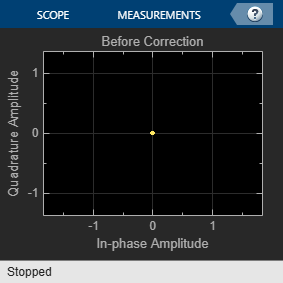The error rate for the demodulated signal without AGC is primarily caused by free space path loss and I/Q imbalance. The QPSK modulation minimizes the effects of the other impairments.

```Error rate for corrected signal: 0.000 Error rate for uncorrected signal: 0.042 ```

To explore the model try:

• Adjusting RF impairment settings, rerun the model, and notice the changes to the constellation diagrams and error rates.

• Modifying the model to add an equalizer stage before the demodulation. Equalization has inherent ability to reduce some of the distortion caused by impairments. For more information, see Equalization.

## Ports

### Input

expand all

Input RF baseband signal, specified as a scalar or column vector.

Data Types: `double` | `single`
Complex Number Support: Yes

### Output

expand all

Output RF baseband signal, returned as a scalar or column vector. The output is of the same data type as the input.

## Parameters

expand all

To edit block parameters interactively, use the Property Inspector. From the Simulink® Toolstrip, on the Simulation tab, in the Prepare gallery, select .

### Main Tab

Specify the amplifier nonlinearity model as one of the following methods:

• `Cubic polynomial`

• `AM/AM - AM/PM`

• `Modified Rapp`

• `Saleh`

Linear gain, specified as a scalar in dB.

#### Dependencies

To enable this parameter, set Model to ```Cubic polynomial``` or ```Modified Rapp```.

Third-order nonlinearity type in dBm, specified as one of these options:

• `IIP3` — Input third-order intercept point

• `OIP3` — Output third-order intercept point

• `IP1dB` — Input 1 dB compression point

• `OP1dB` — Output 1 dB compression point

• `IPsat` — Input saturation point

• `OPsat` — Output saturation point

#### Dependencies

To enable this parameter, set Model to ```Cubic polynomial```.

Input third-order intercept point, specified as a real positive number in dBm.

#### Dependencies

To enable this parameter, set Model to ```Cubic polynomial``` and Type of Non-Linearity to `IIP3`.

Output third-order intercept point, specified as a real positive number in dBm.

#### Dependencies

To enable this parameter, set Model to ```Cubic polynomial``` and Type of Non-Linearity to `OIP3`.

Input 1 dB compression point, specified as a real positive number in dBm.

#### Dependencies

To enable this parameter, set Model to ```Cubic polynomial``` and Type of Non-Linearity to `IP1dB`.

Output 1 dB compression point, specified as a real positive number in dBm.

#### Dependencies

To enable this parameter, set Model to ```Cubic polynomial``` and Type of Non-Linearity to `OP1dB`.

Input saturation point, specified as a real positive number in dBm.

#### Dependencies

To enable this parameter, set Model to ```Cubic polynomial``` and Type of Non-Linearity to `IPsat`.

Output saturation point, specified as a positive real number in dBm.

#### Dependencies

To enable this parameter, set Model to ```Cubic polynomial``` and Type of Non-Linearity to `OPsat`.

Table lookup entries specified as a real M-by-3 matrix. This table expresses the model output power dBm level in matrix column 2 and the model phase change in degrees in matrix column 3 as related to the absolute value of the input signal power of matrix column 1 for the AM/AM-AM/PM model method. The column 1 input power must increase monotonically.

#### Dependencies

To enable this parameter, set Model to ```AM/AM - AM/PM```.

Voltage output saturation level, specified as a real positive number in dBm.

#### Dependencies

To enable this parameter, set Model to ```Modified Rapp```.

Magnitude smoothness factor for the AM/AM calculations of the modified Rapp model method, specified as a positive real number.

#### Dependencies

To enable this parameter, set Model to ```Modified Rapp```.

Phase gain for the AM/PM calculations of the modified Rapp model method, specified as a real scalar in radians.

#### Dependencies

To enable this parameter, set Model to ```Modified Rapp```.

Phase saturation for the AM/PM calculations of the modified Rapp model method, specified as a positive real number.

#### Dependencies

To enable this parameter, set Model to ```Modified Rapp```.

Phase smoothness factor for the AM/PM calculations of the modified Rapp model method, specified as a positive real number.

#### Dependencies

To enable this parameter, set Model to ```Modified Rapp```.

Scaling factor for input signal level for the Saleh model method, specified as a nonnegative real number in dB.

#### Dependencies

To enable this parameter, set Model to `Saleh`.

AM/AM two-tuple conversion parameters for the Saleh model method, specified as a two-element vector of nonnegative real numbers.

#### Dependencies

To enable this parameter, set Model to `Saleh`.

AM/PM two-tuple conversion parameters for the Saleh model mehtod, specified as a two-element vector of nonnegative real numbers.

#### Dependencies

To enable this parameter, set Model to `Saleh`.

Scaling factor for output signal level for the Saleh model method, specified as nonnegative real number in dB.

#### Dependencies

To enable this parameter, set Model to `Saleh`.

Reference load value in ohms, specified as a positive scalar. Use this value to convert between the voltage levels and the signal and noise power levels.

Tunable: Yes

This button plots the power characteristics based on the parameters specified on the Model tab.

Type of simulation to run, specified as `Code generation` or `Interpreted execution`.

• `Code generation` — Simulate the model by using generated C code. The first time you run a simulation, Simulink generates C code for the block. The model reuses the C code for subsequent simulations unless the model changes. This option requires additional startup time, but the speed of the subsequent simulations is faster than with the `Interpreted execution` option.

• `Interpreted execution` — Simulate the model by using the MATLAB® interpreter. This option shortens startup time, but the speed of subsequent simulations is slower than with the ```Code generation``` option. In this mode, you can debug the source code of the block.

## Block Characteristics

 Data Types `double` | `single` Multidimensional Signals `no` Variable-Size Signals `no`

expand all

## Tips

• To visualize the power characteristics of your model, set the parameters listed in the table and click the Plot power characteristics button.

ModelParameters and Example ValuesPower Characteristics Plot
Cubic Polynomial

Main tab:

• Linear power gain (dB): `7`

• Type of Non-linearity: `IIP3`

• IIP3 (dBm): `33`

• Simulate using: `Code generation`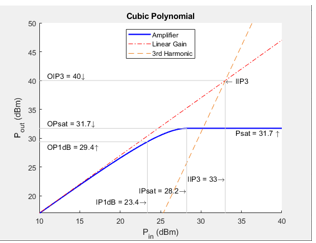AM/AM - AM/PM

Main tab:

• Lookup table (Pin(dBm), Pout(dBm), deg): ```[-25, 5, -1; -10, 20, -2; 0, 27, 5; 5, 28, 12]```

• Simulate using: `Code generation`Modified Rapp

Main tab:

• Linear power gain (dB): `7`

• Output saturation level (V): `1`

• Magnitude smoothness factor: `2`

• Phase gain (rad): `-.45`

• Phase saturation: `0.88`

• Phase smoothness factor: `3.43`

• Simulate using: `Code generation`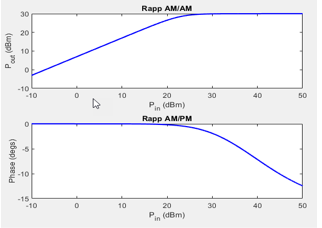Saleh

Main tab:

• Input scaling (dB): `0`

• AM/AM parameters [alpha beta]: ```[ 2.1587, 1.1517 ]```

• AM/PM parameters [alpha beta]: ```[ 4.0033, 9.1040 ]```

• Output scaling (dB): `0`

• Simulate using: ```Interpreted execution```Saleh, A.A.M. “Frequency-Independent and Frequency-Dependent Nonlinear Models of TWT Amplifiers.” IEEE Transactions on Communications 29, no. 11 (November 1981): 1715–20. https://doi.org/10.1109/TCOM.1981.1094911.

 Ghorbani, A., and M. Sheikhan. "The Effect of Solid State Power Amplifiers (SSPAs) Nonlinearities on MPSK and M-QAM Signal Transmission." In 1991 Sixth International Conference on Digital Processing of Signals in Communications, 193–97, 1991.

 Rapp, Ch. "Effects of HPA-Nonlinearity on a 4-DPSK/OFDM-Signal for a Digital Sound Broadcasting System." In Proceedings Second European Conf. on Sat. Comm. (ESA SP-332), 179–84. Liege, Belgium, 1991. https://elib.dlr.de/33776/.

 Kundert, Ken."Accurate and Rapid Measurement of IP2 and IP3," The Designer Guide Community, May 22, 2002.